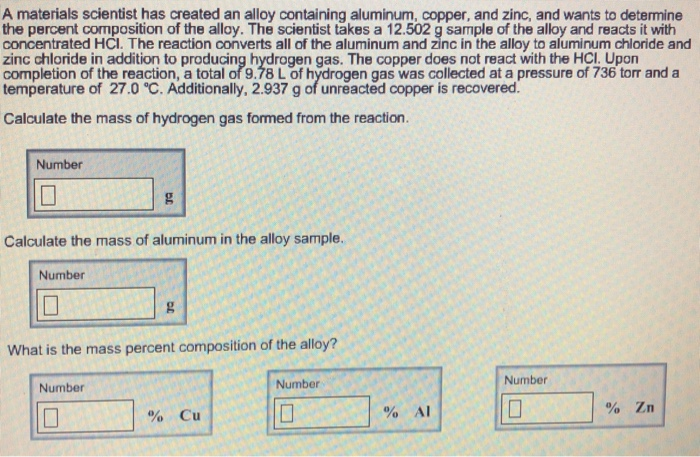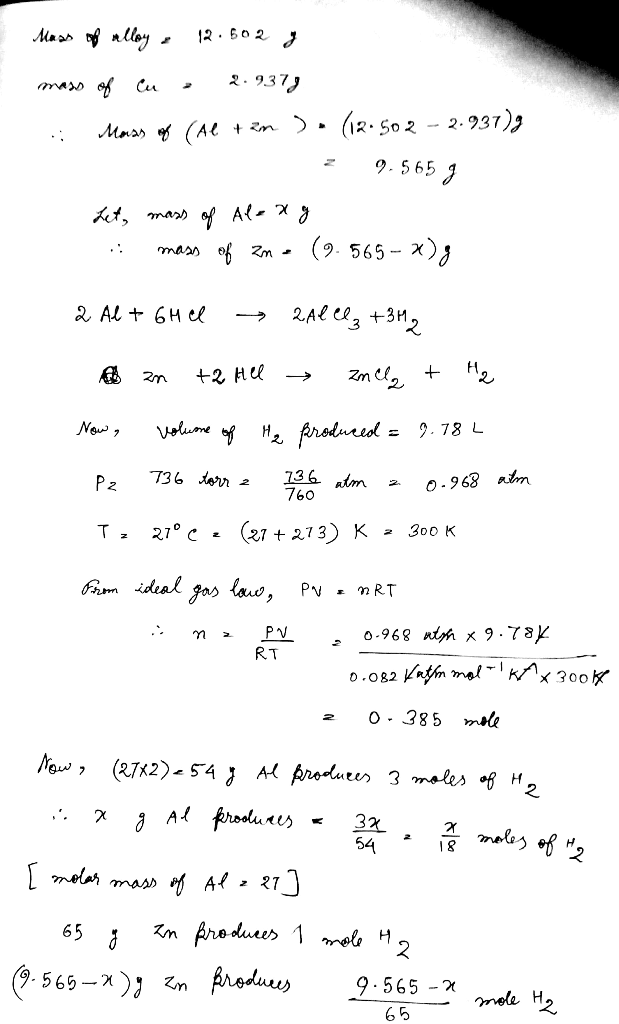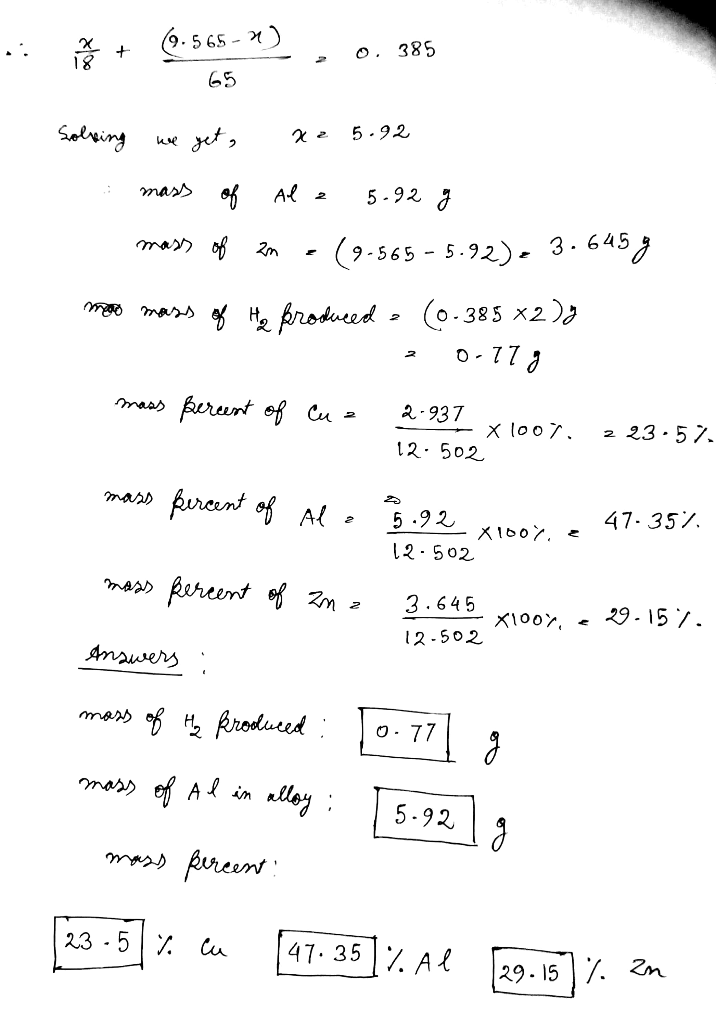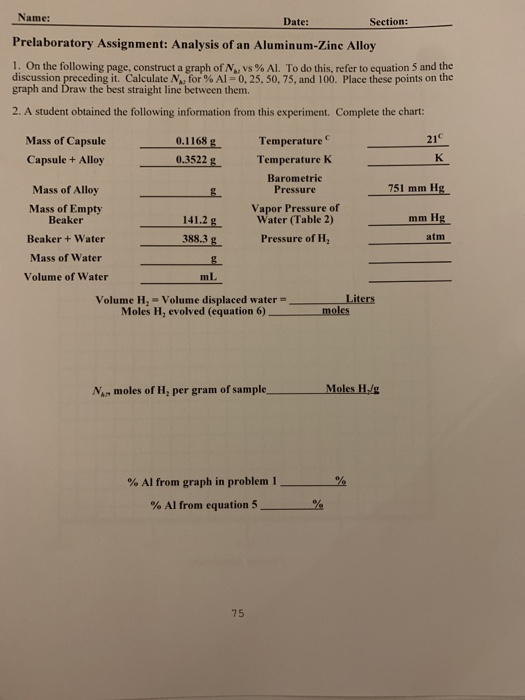Question#### Earn Coins

Coins can be redeemed for fabulous gifts.

Similar Homework Help Questions
• ### Prelaboratory Assignment: Analysis of an Aluminum-Zinc Alloy having a difficult time figuring out how to tackle...Prelaboratory Assignment: Analysis of an Aluminum-Zinc Alloy having a difficult time figuring out how to tackle the following: please help! thank you. equation 5 is included in here Name: Date: Section: Prelaboratory Assignment: Analysis of an Aluminum-Zinc Alloy 1. On the following page, construct a graph of N vs % AL. To do this, refer to equation 5 and the discussion preceding it. Calculate for % Al=0.25.50.75, and 100. Place these points on the graph and Draw the best straight...